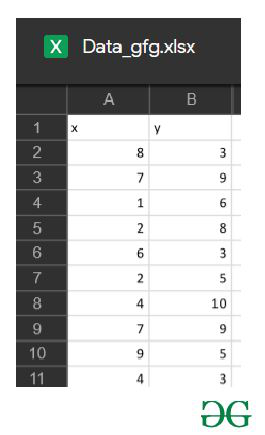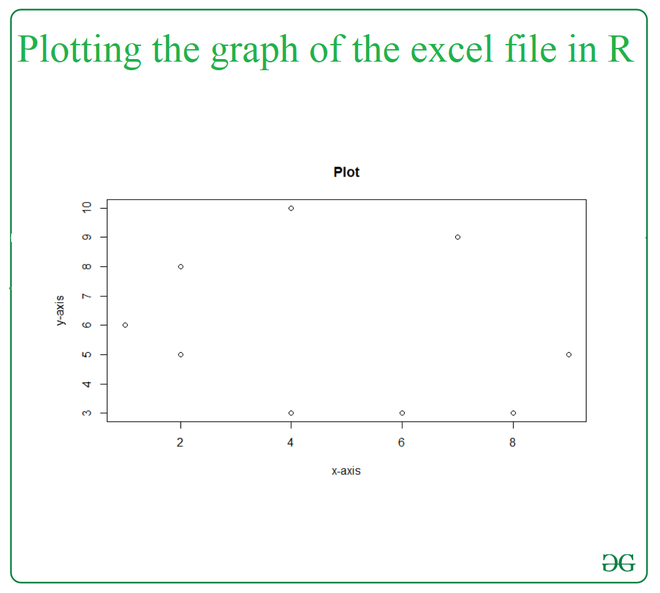# How to plot excel data in R?

• Last Updated : 26 Mar, 2021

Plotting graph in R using an excel file, we need an excel file with two-column in it, the values in the first column will be considered as the points at the x-axis and the values in the second column will be considered as the points at the y-axis. In this article, we will be discussing the approach to plot a graph using an excel file in R language.

To import and read the excel file to the R console, the  read_excel() function from readxl library in R will be used. This function will read an excel-file available in your current working directory. To install the package of the readxl library in R the user must need to follow the following syntax in the R console.

Syntax:

Data in use:plot() function is used for plotting of R objects. With the provided parameters this function returns a scatter plot by default.

Syntax:

plot(x,y,main,xlab,ylab,sub,asp)

Parameters:

• x:-the x coordinates of points in the plot
• y:-the y coordinates of points in the plot
• main:-an overall title for the plot
• sub:-a subtitle for the plot
• xlab:-a title for the x-axis
• ylab:-a title for the y-axis
• asp:-the y/x aspect ratio

Return:

Scatter plot of the given x and y values.

Approach

• Import library
• Import excel file
• Plot graph
• Display plot

Example:

## R

 `library``(readxl)`` ` `Data_gfg <- ``read_excel``(``"Data_gfg.xlsx"``)`` ` `plot``(x = Data_gfg\$x,y = Data_gfg\$y,``     ``xlab = ``"x-axis"``,``     ``ylab = ``"y-axis"``,``     ``main = ``"Plot"``)`

Output:My Personal Notes arrow_drop_up